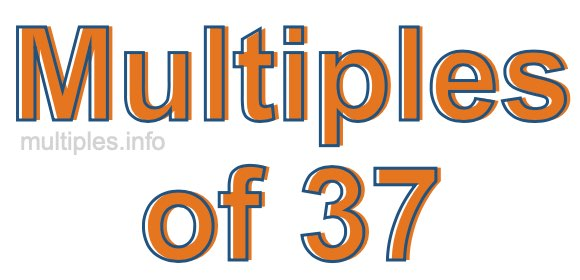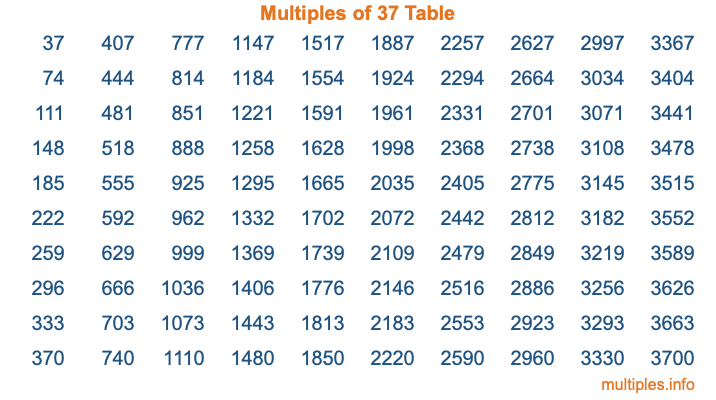Multiples of 37Welcome to the Multiples of 37 page. Here we will first teach you everything you will ever need to know about the multiples of 37, and then give you a study guide summary of everything we taught you to make sure you remember it all. Use this page to look up facts and learn information about the multiples of 37. This page will make you a multiples of thirty-seven expert!

Definition of Multiples of 37
Multiples of 37 are all the numbers that when divided by 37 equal an integer. Each of the multiples of 37 are called a multiple. A multiple of 37 is created by multiplying 37 by an integer.

Therefore, to create a list of multiples of 37, you start with 1 multiplied by 37, then 2 multiplied by 37, then 3 multiplied by 37, and so on for as long as you want. Thus, the list of the first five multiples of 37 is 37, 74, 111, 148, and 185. To see a larger list of multiples of 37, see the printable image of Multiples of 37 further down on this page. We also have a category where you can choose any nth multiple of 37.

Multiples of 37 Checker
The Multiples of 37 Checker below checks to see if any number of your choice is a multiple of 37. In other words, it checks to see if there is any number (integer) that when multiplied by 37 will equal your number. To do that, we divide your number by 37. If the the quotient is an integer, then your number is a multiple of 37.

Is  a multiple of 37?

Least Common Multiple of 37 and ...
A Least Common Multiple (LCM) is the lowest multiple that two or more numbers have in common. This is also called the smallest common multiple or lowest common multiple and is useful to know when you are adding our subtracting fractions. Enter one or more numbers below (37 is already entered) to find the LCM.

Check out our LCM Calculator if you need more details about the Least Common Multiple or if you need the LCM for different numbers for adding and subtraction fractions.

nth Multiple of 37
As we stated above, 37 is the first multiple of 37, 74 is the second multiple of 37, 111 is the third multiple of 37, and so on. Enter a number below to find the nth multiple of 37.

th multiple of 37

Multiples of 37 vs Factors of 37
37 is a multiple of 37 and a factor of 37, but that is where the similarities end. All postive multiples of 37 are 37 or greater than 37. All positive factors of 37 are 37 or less than 37.

Below is the beginning list of multiples of 37 and the factors of 37 so you can compare:

Multiples of 37: 37, 74, 111, 148, 185, etc.

Factors of 37: 1, 37

As you can see, the multiples of 37 are all the numbers that you can divide by 37 to get a whole number. The factors of 37, on the other hand, are all the whole numbers that you can multiply by another whole number to get 37.

It's also interesting to note that if a number (x) is a factor of 37, then 37 will also be a multiple of that number (x).

Multiples of 37 vs Divisors of 37
The divisors of 37 are all the integers that 37 can be divided by evenly. Below is a list of the divisors of 37.

Divisors of 37: 1, 37

The interesting thing to note here is that if you take any multiple of 37 and divide it by a divisor of 37, you will see that the quotient is an integer.

Multiples of 37 Table
Below is an image of the first 100 multiples of 37 in a table. The table is in chronological order, column by column. The first column has the first ten multiples of 37, the second column has the next ten multiples of 37, and so on.The Multiples of 37 Table is also referred to as the 37 Times Table or Times Table of 37. You are welcome to print out our table for your studies.

Negative Multiples of 37
Although not often discussed or needed in math, it is worth mentioning that you can make a list of negative multiples of 37 by multiplying 37 by -1, then by -2, then by -3, and so on, to get the following list of negative multiples of 37:

-37, -74, -111, -148, -185, etc.

Multiples of 37 Summary
Below is a summary of important Multiples of 37 facts that we have discussed on this page. To retain the knowledge on this page, we recommend that you read through the summary and explain to yourself or a study partner why they hold true.

There are an infinite number of multiples of 37.

A multiple of 37 divided by 37 will equal a whole number.

37 divided by a factor of 37 equals a divisor of 37.

The nth multiple of 37 is n times 37.

The largest factor of 37 is equal to the first positive multiple of 37.

37 is a multiple of every factor of 37.

37 is a multiple of 37.

A multiple of 37 divided by a divisor of 37 equals an integer.

37 divided by a divisor of 37 equals a factor of 37.

Any integer times 37 will equal a multiple of 37.

Multiples of a Number
Here you can get the multiples of another number, all with the same attention to detail as we did for multiples of 37 on this page.

Multiples of
Multiples of 38
Did you find our page about multiples of thirty-seven educational? Do you want more knowledge? Check out the multiples of the next number on our list!